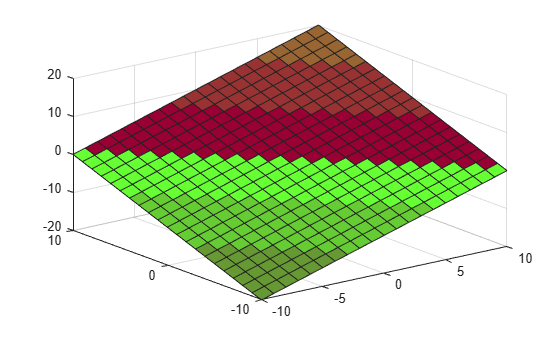# colorcube

Colorcube colormap array

•## Syntax

``c = colorcube``
``c = colorcube(m)``

## Description

example

````c = colorcube` returns the colorcube colormap as a three-column array with the same number of rows as the colormap for the current figure. If no figure exists, then the number of rows is equal to the default length of 256. Each row in the array contains the red, green, and blue intensities for a specific color. The intensities are in the range [0,1], and the color scheme looks like this image.```

example

````c = colorcube(m)` returns the colormap with `m` colors.```

## Examples

collapse all

After getting the colormap array, you can isolate a section of it to use as the color scheme.

Create a surface plot of a plane.

```[X,Y] = meshgrid(-10:1:10); Z = X + Y; surf(X,Y,Z);```Get the colorcube colormap array, and isolate the red section (entries 39 to 44). Then use this section to color the whole surface.

```c = colorcube; c = c(39:44,:); colormap(c);```Create a surface plot of a plane.

```[X,Y] = meshgrid(-10:1:10); Z = X + Y; surf(X,Y,Z);```Get the colorcube colormap with 75 entries. Then use it to color the plane.

```c = colorcube(75); colormap(c);```## Input Arguments

collapse all

Number of colors, specified as a positive number. The default value of `m` is the number of colors in the current figure's colormap. If no figure exists, the default value is 256.

Data Types: `single` | `double`

## Version History

Introduced before R2006a

expand all